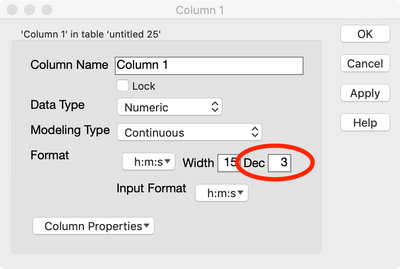Choose Language Hide Translation Bar
Highlighted

## Convert Character Date Time to Date Time

Having difficulty converting to a numeric date time.

JMP log error: Column "Date-Time" changed to type Character to match formula.

Date::Time original format:

01:28:2018::02:14:08:099

What I am trying to do (after many attempts from various posts):

``````dt<< new column ("Date-Time", numeric, formula(
rawdate = substitute(trim(substr(:TimeStamp,1,10)),":","/");
rawtime = (trim(substr(:TimeStamp,13,12)));
datetme = rawdate || " " || rawtime;
//informat(datetime);
//as date(datetme);
)
) ;``````

Informat() and as date() give me "." result

1 ACCEPTED SOLUTION

Accepted Solutions
Highlighted

## Re: Convert Character Date Time to Date Time

Here is the conversion formula I came up with

``````Date MDY(
Num( Substr( :TimeStamp, 1, 2 ) ),
Num( Substr( :TimeStamp, 4, 2 ) ),
Num( Substr( :TimeStamp, 7, 4 ) )
)
+ 3600 * Num( Substr( :TimeStamp, 13, 2 ) )
+ 60 * Num( Substr( :TimeStamp, 16, 2 ) )
+Num( Substr( :TimeStamp, 19, 2 ) )``````

and I am specifying a format of     m/d/y h:m:s    for the new column

Jim
8 REPLIES 8
Highlighted

## Re: Convert Character Date Time to Date Time

Here is the conversion formula I came up with

``````Date MDY(
Num( Substr( :TimeStamp, 1, 2 ) ),
Num( Substr( :TimeStamp, 4, 2 ) ),
Num( Substr( :TimeStamp, 7, 4 ) )
)
+ 3600 * Num( Substr( :TimeStamp, 13, 2 ) )
+ 60 * Num( Substr( :TimeStamp, 16, 2 ) )
+Num( Substr( :TimeStamp, 19, 2 ) )``````

and I am specifying a format of     m/d/y h:m:s    for the new column

Jim
Highlighted

## Re: Convert Character Date Time to Date Time

That worked flawlessly. I added to the column properties in the script: continuous, format("m/d/y h:m:s").

I am not a software engineer or related, just trying to hack my way through this. I guess I don't understand how date and time work in JMP.  The time stamp does not need to be in "MM/DD/YYYY hh:mm:ss:msc" format? From what I found in other posts, I thought that was the correct format when converting from character to date/time.  I also see that you grab each number alone and then change the type, I am assuming that JMP could not handle the character inputs mixed in with the numerics?

Thank you very much again for your help.  Much appreciated.

Highlighted

## Re: Convert Character Date Time to Date Time

Can you please supply a sample of your character string date time column. I am assuming that all you have to do is to strip off the seconds and milliseconds, convert that string to a numeric, and then add that to calculation of the JMP Date/Time value.
Jim
Highlighted

## Re: Convert Character Date Time to Date Time

a quick question here;
If we want to keep the last three digits of decimals for sec (miliseconds) in time format, how do we keep it in formula?
Thanks much from newbie.
HighlightedJeff_PerkinsonCommunity Manager

## Re: Convert Character Date Time to Date Time

As @txnelson says, we could use some more detail and an example but it may be that you just need to specify the number of decimals to display in the format of your column.-Jeff
Highlighted

## Re: Convert Character Date Time to Date Time

Hello,

I reviewed several posts to address this same issue, and am still running into the column getting converted back to character "to match the formula" , per comments in the log file.

I have a table with a Date column "New Date" in string format with entries in the format "MM/DD/YYYY"

I tried to apply your solution to this column:

``````dt << New Column ("Date2", Numeric, "Continuous",
Formula ( Date MDY(Num( Substr( :New_Date, 1, 2 ) ),
Num( Substr( :New_Date, 4, 2 ) ),
Num( Substr( :New_Date, 7, 4 ) ))));``````

Which creates a numeric date column with the standard JMP date format in seconds (e.g. "3651955200") for 9/22/2019.

I then created another column to convert it to a standard date format:

``dt << New Column ("Date3", Numeric, "Continuous", Formula ( Abbrev Date(Date2)));``

But running this just converted the data back to character format when I ran it...

`Column "Date3" changed to type Character to match formula.`

Can you help with the correct conversion procedure?

I am running out of options to try...

-Jens

Highlighted

## Re: Convert Character Date Time to Date Time

What you are missing is the understanding of using a Format to instruct JMP to take the numeric Date value and display it in a Date Format.  A modification of your JSL will show you the simple change to make your code work.

``````dt << New Column( "Date2",
Numeric,
"Continuous",
Formula(
Date MDY(
Num( Substr( :New_Date, 1, 2 ) ),
Num( Substr( :New_Date, 4, 2 ) ),
Num( Substr( :New_Date, 7, 4 ) )
)
),
Format( "m/d/y", 10)
);``````
Jim
Highlighted